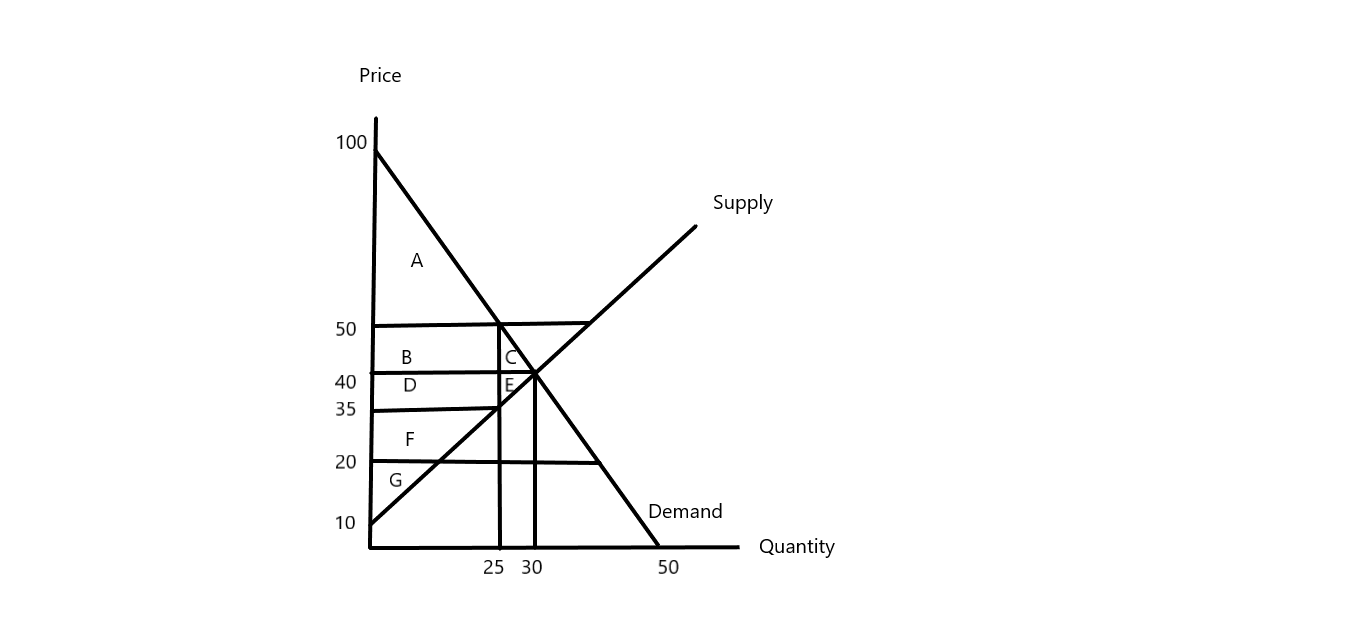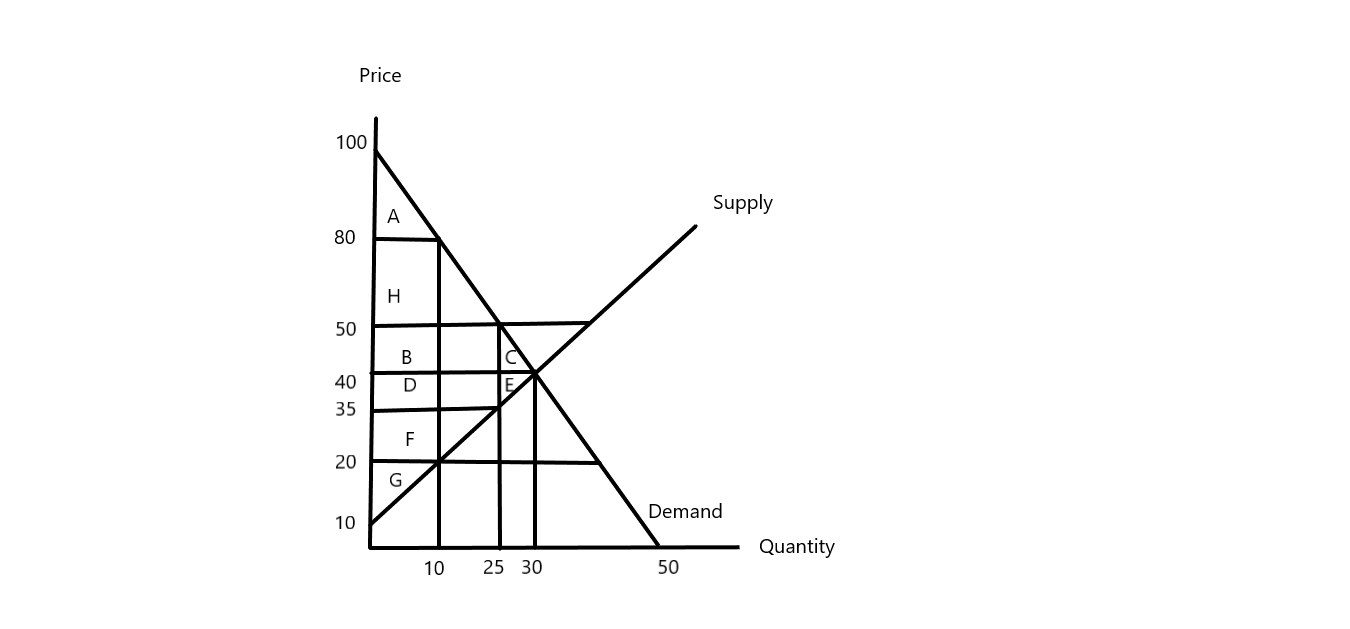In: Economics

# Consider the following supply and demand equations: Supply: p = 10 + q Demand: p =...

Consider the following supply and demand equations: Supply: p = 10 + q Demand: p = 100 − 2q Show your work as your respond to the following questions.

(a) What is the market equilibrium price and quantity?

(b) What is the Total Surplus at equilibrium?

(c) The government enacts a price ceiling at ¯p = 50. What is the Total Surplus?

(d) Calculate the Consumer Surplus under a price ceiling of ¯p = 20.

(e) What is the Deadweight Loss under a price ceiling of ¯p = 10?

## Solutions

##### Expert Solution

Given demand curve is : p=100-2q. When p=0,q=50 and when q=0,p=100. Thus, the demand curve has a vertical intercept of 100 and a horizontal intercept of 50.

Again, supply curve is : p=10+q. When q=0,p=10 and when q=50,p=60. Thus, the supply curve is a straight line that passes through the points (0,10) and (50,60).

In the diagram below, we have plotted the demand and supply curves as :a) Equilibrium is attained at the point where Demand = Supply

or, 100-2q = 10+q

or, 3q = 90

or, q = 30 units is the equilibrium quantity

and p = 100-(2*30) = $40 is the equilibrium price b) Total surplus = consumer surplus Area (A+B+C) + producer surplus Area (D+E+F+G) or, Total surplus = {1/2*(100-40)*30} + {1/2*(40-10)*30} or, Total surplus = (1/2*60*30) + (1/2*30*30) or, Total surplus = 900+450 or, Total surplus =$1,350

c) When there is a price ceiling at p=50, quantity demanded q = 25 units.

Then, consumer surplus Area (A) = 1/2*(100-50)*25 = $625 and producer surplus Area (B+D+F+G) = 1/2*(40+15)*25 =$687.50

Then, total surplus = $1,312.50d) Under price ceiling of p=20, quantity supplied = 10 units Then, consumer surplus = Area (A+H+B+D+F) or, consumer surplus = 1/2*(60+80)*10 or, consumer surplus =$700

e) Under a price ceiling of p=10, quantity supplied = 0

Thus, total surplus = 0

##### Consider the following demand and supply equations in the market for labour. Supply: W = 10...
Consider the following demand and supply equations in the market for labour. Supply: W = 10 + (1/3) L Demand: W = 1,000 − (2/3) L Show your work as you respond to the following questions. What is the market equilibrium wage and quantity? (5%) The government implements a minimum wage of W = 370. What is the Consumer Surplus? (5%) Calculate the Producer Surplus under a minimum wage of W = 370. (5%) Find the Deadweight Loss under a...
##### Consider the following demand and supply equations in the market for labour. Supply: W = 10...
Consider the following demand and supply equations in the market for labour. Supply: W = 10 + (1/3)L Demand: W = 1, 000 − (2/3)L Show your work as you respond to the following questions. (a) What is the market equilibrium wage and quantity? (b) The government implements a minimum wage of W = 370. What is the Consumer Surplus? (c) Calculate the Producer Surplus under a minimum wage of W = 370. (d) Find the Deadweight Loss under a...
##### 1: Assume the following demand and supply equations: Demand: Q = 480 - 35P Supply: Q...
1: Assume the following demand and supply equations: Demand: Q = 480 - 35P Supply: Q = 200 +16P What is the equilibrium Price? What is the equilibrium quantity? 2: Define the four product markets with at least one example of each example. How is the oligopoly market different from the other three types of market?
##### 1: Assume the following demand and supply equations: Demand: Q = 480 - 35P Supply: Q...
1: Assume the following demand and supply equations: Demand: Q = 480 - 35P Supply: Q = 200 +16P What is the equilibrium Price? What is the equilibrium quantity? 2: Define the four product markets with at least one example of each example. How is the oligopoly market different from the other three types of market?